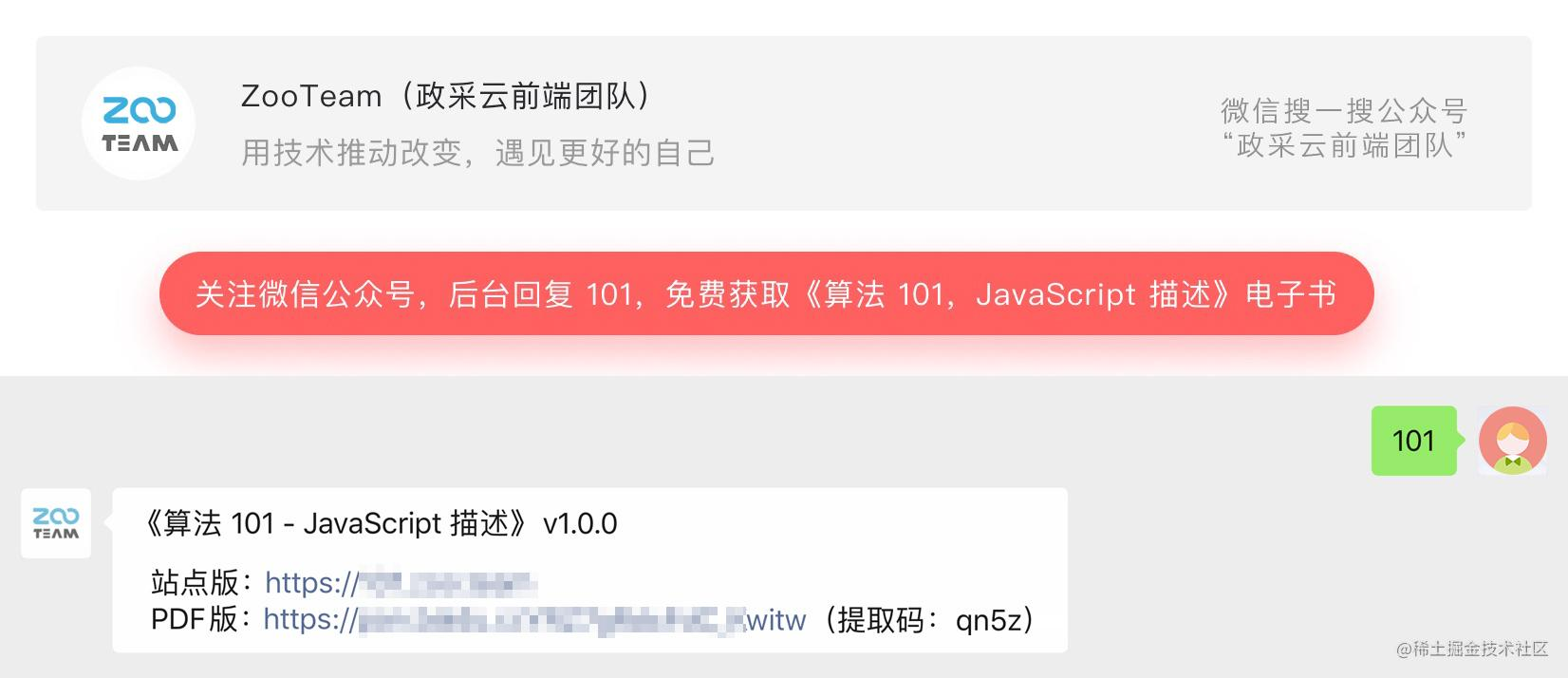# 结合React源码，五分钟带你掌握优先队列公众号 @ 政采云前端团队## 优先队列的操作

• 插入：在优先队列中插入元素，并使队列“有序”
• 删除最大/最小值：删除并返回最大/最小的元素，并使队列“有序”
• 查找最大/最小关键字：查找最大/最小的值

## 二叉堆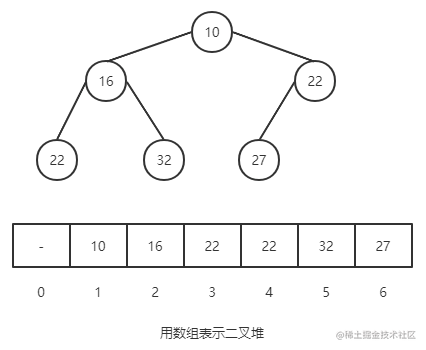• 节点 k 的父节点下标为 k / 2（向下取整）
• 已某节点为根节点的子树，该节点是这颗树的极值

## 二叉堆的操作

### 插入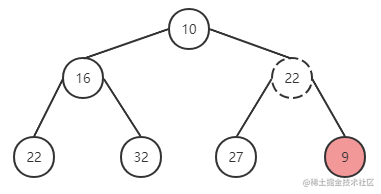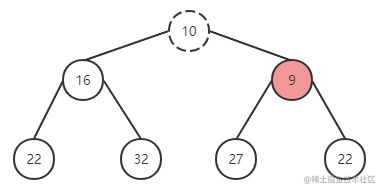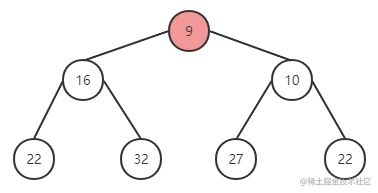#### 程序框架

``````function push {
* 在堆尾部添加元素
* 执行上浮循环
* 与父元素对比大小，将较大的放在父节点位置

return minItem
}

#### 实现

``````function push(heap: Heap, node: Node): void {
const index = heap.length;
heap.push(node); // 在堆尾部添加元素
siftUp(heap, node, index); // 进行上浮操作
}

function siftUp(heap, node, i) {
let index = i;
while (true) {
const parentIndex = (index - 1) >>> 1; // 父节点位置： parentIndex = childIndex / 2
const parent = heap[parentIndex];
if (parent !== undefined && compare(parent, node) > 0) {
// The parent is larger. Swap positions.
heap[parentIndex] = node;
heap[index] = parent;
index = parentIndex;
} else {
// The parent is smaller. Exit.
return;
}
}
}

### 删除

1. 取出根节点的值
2. 将最后一个元素与根节点进行替换，并删除最后一个元素
3. 下沉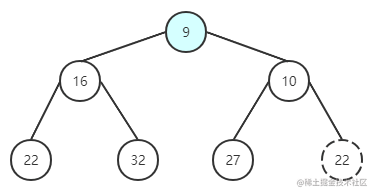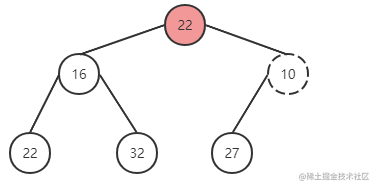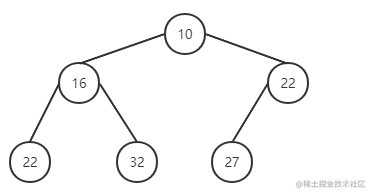#### 程序框架

``````function pop {
* 设定 minItem 保存根节点
* 取出最后一个节点与根节点替换，并删除最后一个节点
* 执行下沉循环
* 将根元素与左右子节点对比,挑选较小的与父节点替换位置

return minItem
}

#### 实现

``````export function pop(heap: Heap): Node | null {
const first = heap; // 取出根节点
if (first !== undefined) {
const last = heap.pop(); // 取出最后一位元素，并删除
if (last !== first) {
heap = last; // 与根节点对调
siftDown(heap, last, 0); // 下沉
}
return first;
} else {
return null;
}
}

function siftDown(heap, node, i) {
let index = i;
const length = heap.length;
while (index < length) {
const leftIndex = (index + 1) * 2 - 1;
const left = heap[leftIndex];
const rightIndex = leftIndex + 1;
const right = heap[rightIndex];

// If the left or right node is smaller, swap with the smaller of those.
// 寻找左右儿子较小的那一个替换
if (left !== undefined && compare(left, node) < 0) { //左子节点小于根节点
if (right !== undefined && compare(right, left) < 0) {
heap[index] = right;
heap[rightIndex] = node;
index = rightIndex;
} else {
heap[index] = left;
heap[leftIndex] = node;
index = leftIndex;
}
} else if (right !== undefined && compare(right, node) < 0) { // 左子节点大于根节点，右子节点小于根节点
heap[index] = right;
heap[rightIndex] = node;
index = rightIndex;
} else {
// Neither child is smaller. Exit.
return;
}
}
}

``````/**
*
* LICENSE file in the root directory of this source tree.
*
* @flow strict
*/

// 定义最小堆极其元素，其中 sortIndex 为最小堆对比的 key，若 sortIndex 相同，则对比 id
type Heap = Array<Node>;
type Node = {|
id: number,
sortIndex: number,
|};

// 入队操作，在入队完成之后进行“上浮”
export function push(heap: Heap, node: Node): void {
const index = heap.length;
heap.push(node);
siftUp(heap, node, index);
}

// 查找最大值
export function peek(heap: Heap): Node | null {
const first = heap;
return first === undefined ? null : first;
}

// 删除并返回最大值
export function pop(heap: Heap): Node | null {
const first = heap; // 取出根节点（哨兵）
if (first !== undefined) {
const last = heap.pop(); // 取出最后一位元素，并删除
if (last !== first) { // 头尾并没有对撞
heap = last; // 与根节点对调
siftDown(heap, last, 0); // 下沉
}
return first;
} else {
return null;
}
}

// 上浮，调整树结构
function siftUp(heap, node, i) {
let index = i;
while (true) {
const parentIndex = (index - 1) >>> 1; // 父节点位置： parentIndex = childIndex / 2，此处使用位操作，右移一位
const parent = heap[parentIndex];
if (parent !== undefined && compare(parent, node) > 0) { // 对比父节点和子元素的大小
// The parent is larger. Swap positions.
heap[parentIndex] = node; // 若父节点较大，则更换位置
heap[index] = parent;
index = parentIndex;
} else {
// The parent is smaller. Exit.
return;
}
}
}

// 下沉，调整树结构
function siftDown(heap, node, i) {
let index = i;
const length = heap.length;
while (index < length) {
const leftIndex = (index + 1) * 2 - 1;
const left = heap[leftIndex];
const rightIndex = leftIndex + 1;
const right = heap[rightIndex];

// If the left or right node is smaller, swap with the smaller of those.
// 寻找左右儿子较小的那一个替换
if (left !== undefined && compare(left, node) < 0) {
if (right !== undefined && compare(right, left) < 0) { // 左子节点小于根节点
heap[index] = right;
heap[rightIndex] = node;
index = rightIndex;
} else {
heap[index] = left;
heap[leftIndex] = node;
index = leftIndex;
}
} else if (right !== undefined && compare(right, node) < 0) { // 左子节点大于根节点，右子节点小于根节点
heap[index] = right;
heap[rightIndex] = node;
index = rightIndex;
} else {
// Neither child is smaller. Exit.
return;
}
}
}

function compare(a, b) {
// Compare sort index first, then task id.
const diff = a.sortIndex - b.sortIndex;
return diff !== 0 ? diff : a.id - b.id;
}

## 多叉堆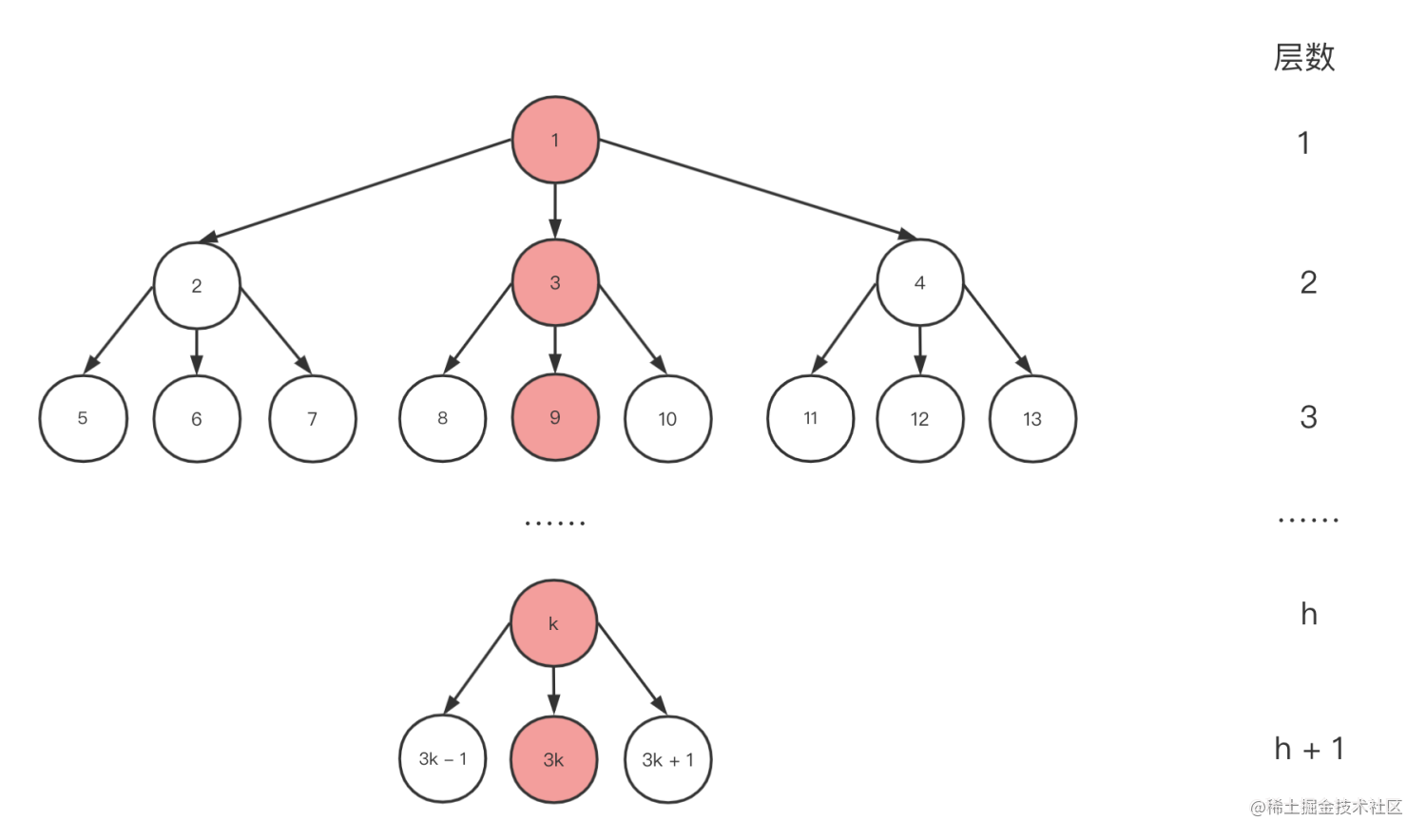``````/*
* at the moment we allow libev the luxury of two heaps,
* a small-code-size 2-heap one and a ~1.5kb larger 4-heap
* which is more cache-efficient.
* the difference is about 5% with 50000+ watchers.
*/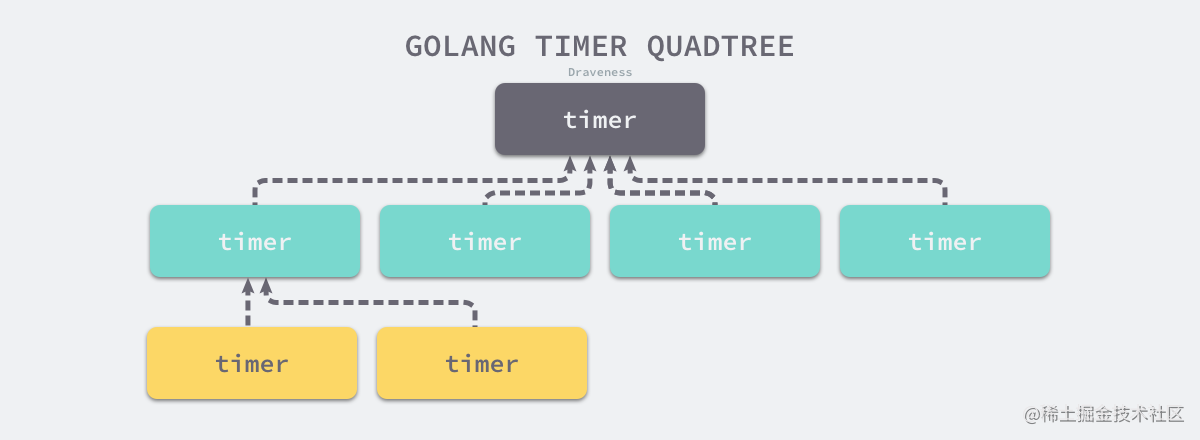## 招贤纳士